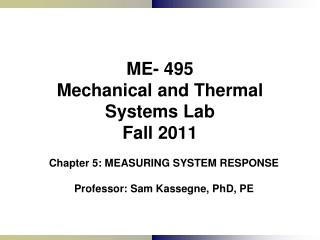DownloadDownload PresentationME- 495 Mechanical and Thermal Systems Lab Fall 2011

ME- 495 Mechanical and Thermal Systems Lab Fall 2011

Download PresentationME- 495 Mechanical and Thermal Systems Lab Fall 2011

- - - - - - - - - - - - - - - - - - - - - - - - - - - E N D - - - - - - - - - - - - - - - - - - - - - - - - - - -
Presentation Transcript

1. ME- 495Mechanical and Thermal Systems LabFall 2011 Chapter 5: MEASURING SYSTEM RESPONSE Professor: Sam Kassegne, PhD, PE

2. Signal Response of Measurand?

3. RESPONSE • System Response: an evaluation of the systems ability to faithfully sense, transmit and present all pertinent information included in the measurand and exclude all else: • Key response characteristics/components are: • Amplitude response • Frequency response • Phase response • Slew Rate

4. COMPONENTS OF SYSTEM RESPONSE 1) Amplitude response: • ability to treat all input amplitudes uniformly • Overdriving – exceeding an amplifiers ability to maintain consistent proportional output • Gain = Amplification = So/Si • Smin<Si<Smax Overloaded in this range.

5. COMPONENTS OF SYSTEM RESPONSE 2) Frequency Response • ability to measure all frequency components proportionally • Attenuation: loss of signal frequencies over a specific range Attenuated in this range.

6. COMPONENTS OF SYSTEM RESPONSE 3) Phase Response • amplifiers ability to maintain the phase relationships in a complex wave. • This is usually important for complex waves unlike amplitude and frequency responses which are important for all types of input wave forms. Why?

7. COMPONENTS OF SYSTEM RESPONSE • 4) Delay/Rise time: time delay between start of step but before proper output magnitude is reached. • Slew rate: maximum rate of change that the system can handle (de/dt) (i.e. for example 25 volts/microsecond)

8. Dynamic Characteristics of Simplified Mechanical Systems Generalized Equation of Motion for a Spring Mass Damper System(1-axis) • F(t) = general excitation force •  = fundamental circular forcing frequency

9. FIRST ORDER SYSTEM(I.A) Step Forced If mass = 0, we get a first-order system. E.g. Temperature sensing systems • F(t)=0 for t<0 • F(t) = F0 for t >= 0 • t=time, k=deflection constant • s=displacement, =damping coefficient • Fo=amplitude of input force • This can be reduced to the general form: (after integration over time and simplification) • P=magnitude of any first order system at time t • P=limiting magnitude of the process as t  • PA=initial magnitude of process at t=0 •  = time constant = /k The above equation could be used to define processes such as a heated/cooled bulk or mass, such as temperature sensor subjected to a step-temperature change, simple capacitive-resistive or inductive-resistive circuits, and the decay of a radioactive source.

10. Figure (a) depicts progressive process Figure (b) depicts decaying process

11. FIRST ORDER SYSTEM(I.B) Harmonically Excited F(t) = Fo cosWt

12. PHASE LAG

13. First Order System – Harmonically Excited – ExampleTemperature Probe Example

14. TEMPERATURE PROBE EXAMPLE - Continued

15. TEMPERATURE PROBE EXAMPLE - Continued

16. (II) SECOND ORDER SYSTEM(II.A) Step Input • Step input • F=0 when t<0 • F=Fo when t>0 • Underdamped Eq:

17. OVERDAMPED SECOND ORDER SYSTEM • =  / C >1 This represents both damped and under-damped cases.

18. (II) SECOND ORDER SYSTEM(II.B) Harmonically Excited F(t) = Fo cosWt

19. MICROPHONE EXAMPLE Second Order System – Harmonically Excited ExampleMicrophone Example

20. MICROPHONE EXAMPLE